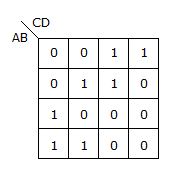# Electronics and Communication Engineering - Exam Questions Papers

6.
i1(t) for t > 0 is given by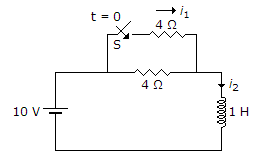2.5 - 2.5e-2t
2.5 - 1.25e-2t
1.25 - 1.25e-2t
None of these
Explanation:

The time constant t is given by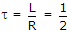As Req = 4 || 4 = 2 Ω

i1(t) = iF - (iF - i1)e-t/t

ifinal =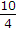= 2.5 A

i1(t) = 2.5 - (2.5 - 1.25)e-t/1/2

i1(t) = 2.5 - 1.25e-2t.

7.
Find I in 4 Ω resistor.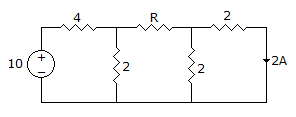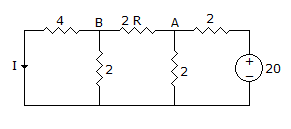1 A
0.5 A
0.75 A
0.95 A
Explanation:

Apply KVL to first to first loop

6i1 - 2i2 = 10

(4 + R)i2 - 2i1 - 2i3 = 0

4i3 - 2i2 = 0

but i3 = 0.5 A

2 - 2i2 = 0

i2 = 1 A, 6i1 - 2 = 10

i1 = 2 A, (4 + R) - 2 X 2 -1 = 0

R = 1 Ω

Using Nodal analysis for loop 2

At node A,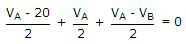VA - 20 + VA + VA - VB = 0

3VA - VB - 20 = 0

3VA - VB = 20 ...(i)

At node B,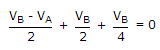2VB - 2VA + 2VB + VB = 0

5VB - 2VA = 0 ...(ii)

Multiplying (i) by 2 and (ii) by 3

6VA - 2VB = 40

- 6VA - 15VB = 0

13VB = 40

VB ≅ 3 V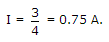8.
Which of the following Boolean Expression correctly represents the relation between P, Q, R and M1?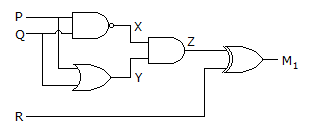M1 = (P OR Q) XOR R
M1 = (P AND Q) XOR R
M1 = (P NOR Q) XOR R
M1 = (P XOR Q) XOR R
Explanation:

M1 = Z XOR R

M1 = (X, Y) XOR R

= M1 = [P.Q.(P + Q)]XOR R

= (PQ + PQ) XOR R = (P XOR Q) XOR R.

9.
A sequential multiplexer is connected as shown in figure. Each time the multiplexer receives the clock, it switches to the next channel from 6 to 1. If the input signals are :
A = 10 cos 2p(4 x 103t)
B = 15 cos 2p(5 x 103t)
C = 20 cos 2p(6 x 103t)
D = 10 cos 2p(10 x 103t)
The minimum clock frequency should be __________ kHz.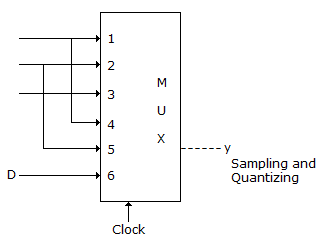8 kHz
50 kHz
20 kHz
10 kHz
Explanation:

Minimum clock freq. = 2fm

Where fm is highest freq. component = 2 x 10 kHz = 10 kHz.

10.
The Boolean function realized by the logic circuit shown is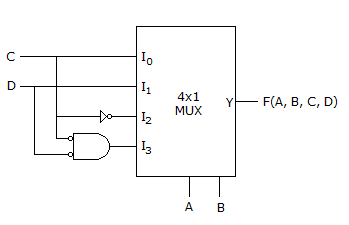F = Σm(0, 1, 3, 5, 9, 10, 14)
F = Σm(2, 3, 5, 7, 8, 12, 13)
F = Σm(1, 2, 4, 5, 11, 14, 15)
F = Σm(2, 3, 5, 7, 8, 9, 12)